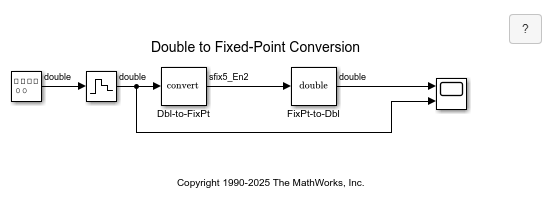# Double to Fixed-Point Conversion

This model shows how to convert signals between built-in and fixed-point data types and illustrates how fixed-point data types affect the representable precision and range. The fixed-point data type used in this model is `fixdt(1,5,2)`, which is a signed 5-bit number with 2 bits to the right of the binary point:

``` Precision = (2^-2) = 0.25 Representable minimum = -(2^-2)*(2^4) = -4 Representable maximum = (2^-2)*(2^4-1) = 3.75```

Open the Data Type Conversion block `Dbl-to-FixPt` to modify the attributes of the fixed-point data type and see how they impact the range and precision of the resulting fixed-point signal.Tips for specifying fixed-point data types:

• Click the `>>` button to open the Data Type Assistant.

• You can specify the output minimum/maximum and use these values to calculate the best-precision scaling, which maximizes the precision while covering the specified range.

• Once you are familiar with the syntax for `fixdt`, you can enter the expression directly into the data type parameter without using the Data Type Assistant.